# GPvecchia tutorial

#### 2022-10-24

In this vignette, we will demonstrate the main capabilities of the GPvecchia package. These include estimating parameters and making spatial predictions. We also show how to use the package for processing non-linear, non-Gaussian data by combining the Vecchia with a Laplace approximation.

We start by importing the GPvecchia library.

library(GPvecchia)
library(Matrix)
library(fields)
#> Spam version 2.6-0 (2020-12-14) is loaded.
#> Type 'help( Spam)' or 'demo( spam)' for a short introduction
#> and overview of this package.
#> Help for individual functions is also obtained by adding the
#> suffix '.spam' to the function name, e.g. 'help( chol.spam)'.
#>
#> Attaching package: 'spam'
#> The following object is masked from 'package:Matrix':
#>
#>     det
#> The following objects are masked from 'package:base':
#>
#>     backsolve, forwardsolve
#> See https://github.com/NCAR/Fields for
#>  an extensive vignette, other supplements and source code

### Simulating data for illustration

To illustrate our method, we simulate a small dataset. First, consider a unit square and randomly select observation locations. (Set spatial.dim=1 to consider data on the one-dimensional unit interval.)

set.seed(1988)
spatial.dim=2
n=50
if(spatial.dim==1){
locs=matrix(runif(n),ncol=1)
} else {
locs <- cbind(runif(n),runif(n))
}

Next, we define the covariance function of the field as well as the scale of the measurement error

beta=2
sig2=1; range=.1; smooth=1.5
covparms =c(sig2,range,smooth)
covfun <- function(locs) sig2*MaternFun(fields::rdist(locs),covparms)
nuggets=rep(.1,n)

We are now ready to simulate the field and visualize it as a sanity check.

Om0 <- covfun(locs)+diag(nuggets)
z=as.numeric(t(chol(Om0))%*%rnorm(n))
data=z+beta

# plot simulated data
if(spatial.dim==1) {
plot(locs,data)
} else {
fields::quilt.plot(locs,data, nx=n, ny=n)
}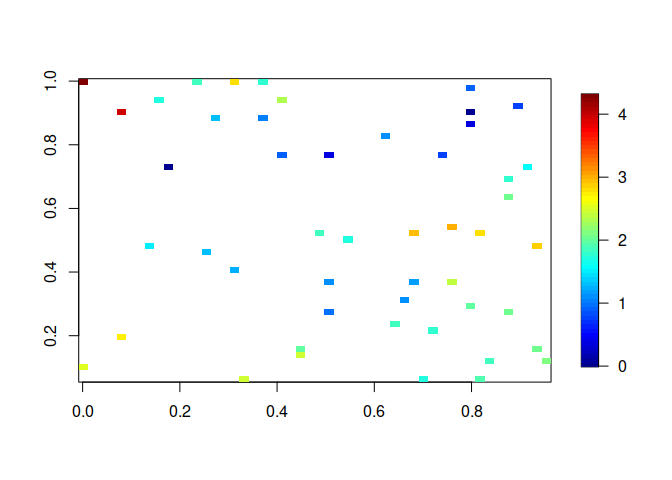We also create a grid of $$n.p$$ locations at which we would like to make predictions.

n.p=100
if(spatial.dim==1){  #  1-D case
locs.pred=matrix(seq(0,1,length=n.p),ncol=1)
} else {   # 2-D case
grid.oneside=seq(0,1,length=round(sqrt(n.p)))
locs.pred=as.matrix(expand.grid(grid.oneside,grid.oneside)) # grid of pred.locs
}
n.p=nrow(locs.pred)

### Basic functions for parameter estimation and prediction

Let us now estimate the mean and covariance parameters using the default settings, which assume a spatially constant mean or trend, and a Matern covariance structure. Note that the following code might take a minute or so to run.

vecchia.est=vecchia_estimate(data,locs,,output.level=0)
#>   Nelder-Mead direct search function minimizer
#> function value for initial parameters = 51.886139
#>   Scaled convergence tolerance is 7.73164e-07
#> Stepsize computed as 0.100000
#> BUILD              5 52.481379 51.745585
#> LO-REDUCTION       7 51.948524 51.745585
#> LO-REDUCTION       9 51.917157 51.745585
#> EXTENSION         11 51.886139 51.685267
#> LO-REDUCTION      13 51.802993 51.685267
#> REFLECTION        15 51.769394 51.677037
#> REFLECTION        17 51.760236 51.657379
#> REFLECTION        19 51.745585 51.628487
#> LO-REDUCTION      21 51.685267 51.628487
#> LO-REDUCTION      23 51.677037 51.628487
#> EXTENSION         25 51.670822 51.613598
#> LO-REDUCTION      27 51.657379 51.613598
#> LO-REDUCTION      29 51.646551 51.613598
#> LO-REDUCTION      31 51.628700 51.612694
#> EXTENSION         33 51.628487 51.589844
#> HI-REDUCTION      35 51.625174 51.589844
#> EXTENSION         37 51.613598 51.580020
#> LO-REDUCTION      39 51.612694 51.580020
#> EXTENSION         41 51.610738 51.551541
#> LO-REDUCTION      43 51.589844 51.551541
#> EXTENSION         45 51.586075 51.543697
#> HI-REDUCTION      47 51.580020 51.543697
#> EXTENSION         49 51.558864 51.500596
#> LO-REDUCTION      51 51.555766 51.500596
#> LO-REDUCTION      53 51.551541 51.500596
#> LO-REDUCTION      55 51.543697 51.500596
#> EXTENSION         57 51.528609 51.471347
#> LO-REDUCTION      59 51.522478 51.471347
#> LO-REDUCTION      61 51.519018 51.471347
#> EXTENSION         63 51.500596 51.459820
#> LO-REDUCTION      65 51.488154 51.459533
#> REFLECTION        67 51.474606 51.445694
#> LO-REDUCTION      69 51.471347 51.445694
#> HI-REDUCTION      71 51.459820 51.445694
#> LO-REDUCTION      73 51.459533 51.445694
#> EXTENSION         75 51.453392 51.435057
#> LO-REDUCTION      77 51.445841 51.435057
#> HI-REDUCTION      79 51.445729 51.435057
#> LO-REDUCTION      81 51.445694 51.435057
#> REFLECTION        83 51.440167 51.431711
#> LO-REDUCTION      85 51.438790 51.431711
#> LO-REDUCTION      87 51.436461 51.431711
#> HI-REDUCTION      89 51.435562 51.431711
#> LO-REDUCTION      91 51.435057 51.431711
#> EXTENSION         93 51.434187 51.428874
#> LO-REDUCTION      95 51.433642 51.428874
#> EXTENSION         97 51.432437 51.425536
#> EXTENSION         99 51.431711 51.420513
#> LO-REDUCTION     101 51.429370 51.420513
#> EXTENSION        103 51.428874 51.419521
#> EXTENSION        105 51.425536 51.410119
#> EXTENSION        107 51.421542 51.401623
#> LO-REDUCTION     109 51.420513 51.401623
#> LO-REDUCTION     111 51.419521 51.401623
#> EXTENSION        113 51.410119 51.388863
#> LO-REDUCTION     115 51.408730 51.388863
#> EXTENSION        117 51.404974 51.378210
#> EXTENSION        119 51.401623 51.371816
#> REFLECTION       121 51.392448 51.371421
#> REFLECTION       123 51.388863 51.371381
#> REFLECTION       125 51.378210 51.366421
#> LO-REDUCTION     127 51.371816 51.366421
#> HI-REDUCTION     129 51.371421 51.366421
#> HI-REDUCTION     131 51.371381 51.366421
#> REFLECTION       133 51.368353 51.364609
#> LO-REDUCTION     135 51.368258 51.364609
#> LO-REDUCTION     137 51.367375 51.364609
#> REFLECTION       139 51.366421 51.363815
#> LO-REDUCTION     141 51.365054 51.363603
#> REFLECTION       143 51.364712 51.363599
#> LO-REDUCTION     145 51.364609 51.363342
#> LO-REDUCTION     147 51.363815 51.363166
#> HI-REDUCTION     149 51.363603 51.363166
#> HI-REDUCTION     151 51.363599 51.363166
#> REFLECTION       153 51.363342 51.363100
#> HI-REDUCTION     155 51.363246 51.363100
#> HI-REDUCTION     157 51.363238 51.363100
#> EXTENSION        159 51.363166 51.362916
#> HI-REDUCTION     161 51.363105 51.362916
#> EXTENSION        163 51.363102 51.362823
#> LO-REDUCTION     165 51.363100 51.362823
#> REFLECTION       167 51.363009 51.362765
#> LO-REDUCTION     169 51.362916 51.362765
#> LO-REDUCTION     171 51.362827 51.362737
#> HI-REDUCTION     173 51.362823 51.362737
#> EXTENSION        175 51.362773 51.362601
#> LO-REDUCTION     177 51.362765 51.362601
#> LO-REDUCTION     179 51.362752 51.362601
#> EXTENSION        181 51.362737 51.362535
#> REFLECTION       183 51.362655 51.362519
#> HI-REDUCTION     185 51.362631 51.362519
#> LO-REDUCTION     187 51.362601 51.362519
#> REFLECTION       189 51.362571 51.362488
#> HI-REDUCTION     191 51.362535 51.362488
#> LO-REDUCTION     193 51.362532 51.362488
#> LO-REDUCTION     195 51.362523 51.362488
#> REFLECTION       197 51.362519 51.362487
#> EXTENSION        199 51.362491 51.362454
#> EXTENSION        201 51.362490 51.362449
#> HI-REDUCTION     203 51.362488 51.362449
#> EXTENSION        205 51.362487 51.362430
#> REFLECTION       207 51.362466 51.362421
#> LO-REDUCTION     209 51.362454 51.362421
#> LO-REDUCTION     211 51.362449 51.362421
#> REFLECTION       213 51.362430 51.362417
#> HI-REDUCTION     215 51.362424 51.362417
#> REFLECTION       217 51.362422 51.362412
#> LO-REDUCTION     219 51.362421 51.362412
#> HI-REDUCTION     221 51.362417 51.362412
#> LO-REDUCTION     223 51.362417 51.362412
#> REFLECTION       225 51.362412 51.362411
#> HI-REDUCTION     227 51.362412 51.362409
#> REFLECTION       229 51.362412 51.362409
#> LO-REDUCTION     231 51.362412 51.362409
#> HI-REDUCTION     233 51.362411 51.362409
#> HI-REDUCTION     235 51.362409 51.362409
#> Exiting from Nelder Mead minimizer
#>     237 function evaluations used

Based on these parameter estimates, we can then make predictions at the grid of locations we had specified above.

preds=vecchia_pred(vecchia.est,locs.pred)

Finally, we compare the approximate predictions with the best possible ones (i.e. those obtained using analytic expressions for conditional mean in the Gaussian distribution).

##  exact prediction
mu.exact=as.numeric(covfun(rbind(locs,locs.pred))[,1:n]%*%solve(Om0,data-beta))+beta
cov.exact=covfun(rbind(locs,locs.pred))-
covfun(rbind(locs,locs.pred))[,1:n]%*%solve(Om0,t(covfun(rbind(locs,locs.pred))[,1:n]))
var.exact=diag(cov.exact)
cov.exact.pred=cov.exact[n+(1:n.p),n+(1:n.p)]

### plot Vecchia and exact predictions
if(spatial.dim==1) {
plot(locs,z)
lines(locs.pred,preds$mean.pred,col='blue') lines(locs.pred,preds$mean.pred-1.96*sqrt(preds$var.pred),col='blue',lty=2) lines(locs.pred,preds$mean.pred+1.96*sqrt(preds$var.pred),col='blue',lty=2) lines(locs.pred,mu.exact[n+(1:n.p)],col='red') lines(locs.pred,mu.exact[n+(1:n.p)]-1.96*sqrt(var.exact[n+(1:n.p)]),col='red',lty=2) lines(locs.pred,mu.exact[n+(1:n.p)]+1.96*sqrt(var.exact[n+(1:n.p)]),col='red',lty=2) } else { sdrange=range(sqrt(c(preds$var.pred,var.exact[n+(1:n.p)])))
defpar = par(mfrow=c(2,3))
fields::quilt.plot(locs,z, nx=sqrt(n.p), ny=sqrt(n.p))
fields::quilt.plot(locs.pred,preds$mean.pred, nx=sqrt(n.p), ny=sqrt(n.p)) fields::quilt.plot(locs.pred,sqrt(preds$var.pred),zlim=sdrange, nx=sqrt(n.p), ny=sqrt(n.p))
fields::quilt.plot(locs,z, nx=sqrt(n.p), ny=sqrt(n.p))
fields::quilt.plot(locs.pred,mu.exact[n+(1:n.p)], nx=sqrt(n.p), ny=sqrt(n.p))
fields::quilt.plot(locs.pred,sqrt(var.exact[n+(1:n.p)]),zlim=sdrange, nx=sqrt(n.p), ny=sqrt(n.p))
par(defpar)
}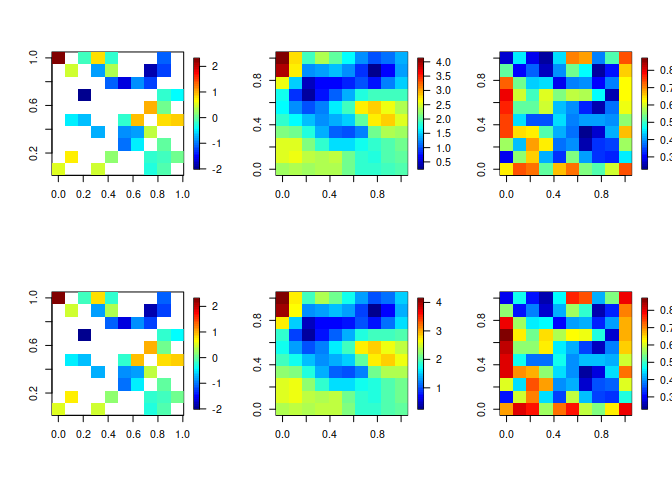### More details on likelihood evaluation

Let’s take a closer look at how the likelihood is evaluated using Vecchia. Most importantly, we can specify a parameter, $$m$$. Its value determines the number of “neighbours” of each point, or, in other words, how many other points a given point conditions on. The larger this parameter, the more accurate and expensive the approximation will be.

m=20
vecchia.approx=vecchia_specify(locs,m)
vecchia_likelihood(z,vecchia.approx,covparms,nuggets)
#>  -51.40362

Note that the function vecchia_specify determines the general properties of the approximation, but it does not depend on the data or the specific parameter values. Hence, it does not have to be re-run when searching over different parameter values in an estimation procedure.

We can also compare the results to the exact likelihood:

library(mvtnorm)
dmvnorm(z,mean=rep(0,n),sigma=Om0,log=TRUE)
#>  -51.441

In this case the approximation is very good. In general, $$m=20$$ is a good value, and $$m$$ should usually be between 10 and 40. For one-dimensional space, we can get good approximations even with $$m=5$$ or smaller.

### More details on spatial prediction

Similar to the previous section we next specify the approximation and indicate at which locations prediction is desired.

m=30
vecchia.approx=vecchia_specify(locs,m,locs.pred=locs.pred)
preds=vecchia_prediction(z,vecchia.approx,covparms,nuggets)
# returns a list with elements mu.pred,mu.obs,var.pred,var.obs,V.ord

It is also possible to print the entire predictive covariance matrix. We do it here only for the purpose of illustration. If $$n.p$$ is very large, this matrix might use up a lot of memory and we generally do not recommend plotting it directly.

Sigma=V2covmat(preds)$Sigma.pred cov.range=quantile(rbind(Sigma,cov.exact.pred),c(.01,.99)) defpar = par(mfrow=c(1,2)) fields::image.plot(cov.exact.pred,zlim=cov.range) fields::image.plot(Sigma,zlim=cov.range)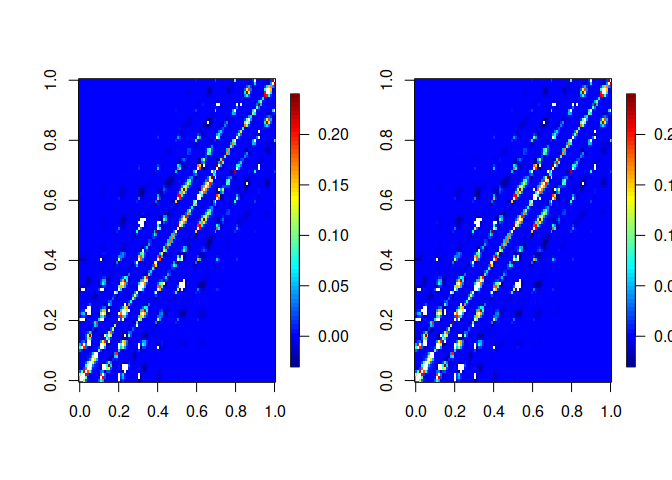par(mfrow=c(defpar)) #### Linear combinations We might sometimes be interested in a linear combination of the predicted values. In particular, we can limit our attention to only a subset of our predictions. This can be accomplished by specifying the linear combination coefficients as a matrix. As an example, we assume we are only interested in predictions at the unobserved prediction locations (not at the first n observed locations): H=Matrix::sparseMatrix(i=1:(n+n.p),j=1:(n+n.p),x=1)[(n+1):(n+n.p),] # compute variances of Hy lincomb.vars=vecchia_lincomb(H,preds$U.obj,preds$V.ord) plot(preds$var.pred,lincomb.vars)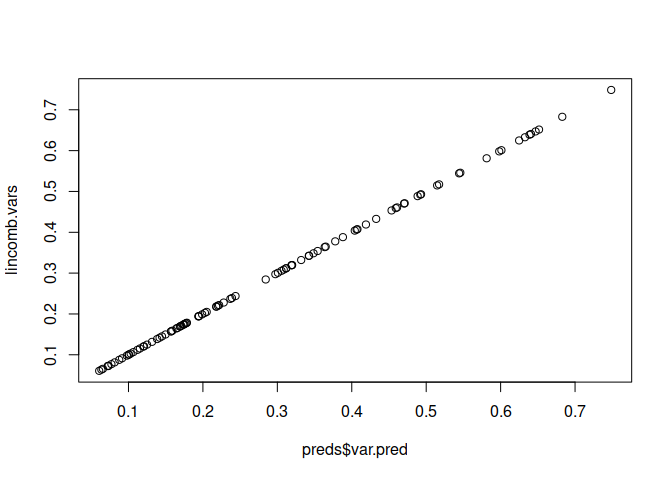As another example, we consider the overall mean of the process at all prediction locations. Using the vecchia_lincomb() function enables us to get the variance estimates easily.

mean(preds$mu.pred) #>  -0.1873878 # compute entire covariance matrix of Hy (here, 1x1) H=Matrix::sparseMatrix(i=rep(1,n.p),j=n+(1:n.p),x=1/n.p) lincomb.cov=vecchia_lincomb(H,preds$U.obj,preds$V.ord,cov.mat=TRUE) ### Other GP approximations as special cases By specifying appropriate options in vecchia_specify, we can do everything described above for several other GP approximations: Modified predictive process, FSA, MRA, latent, standard Vecchia Setting $$M=1$$ results in block full-scale approximation, specifically one with $$r_0 = \frac{m}{2}$$ knots spread over the entire domain and the remaining locations being partitioned into blocks of size $$<\frac{m}{2}+1$$. m=20 mra.options.fulls=list(M=1) blockFS = vecchia_specify(locs, m, 'maxmin', conditioning='mra', mra.options=mra.options.fulls, verbose=TRUE) #> MRA params: m=19; J=4; r=10,10; M=1 Another popular existing approximation method, modified predictive process (MPP), can also be obtained by specifying appropriate parameter settings: mra.options.mpproc=list(r=c(m,1)) MPP = vecchia_specify(locs, m, 'maxmin', conditioning='mra', mra.options=mra.options.mpproc, verbose=TRUE) #> MRA params: m=20; J=32; r=20; M=0 As we can see, MPP can be viewed as a special case of the multi-resolution approximation (MRA). A general MRA is obtained my specifying all of its three parameters mra.options.mra = list(r=c(10, 5, 5), M=2, J=2) MRA_rJM = vecchia_specify(locs, m, 'maxmin', conditioning='mra', mra.options=mra.options.mra, verbose=TRUE) #> Warning in get.mra.params(n, mra.options, m): M, r set for MRA. If parameter m #> was given, it will be overridden #> MRA params: m=22; J=2,2; r=10,5,7; M=2 We should note two things to note about this full specifiction of an MRA. First, providing all three $$r$$,$$J$$ and $$M$$ overrides whatever value of $$m$$ was provided. Second, in order to be able to place a knot at each point of the grid, the provided parameters might need to be adjusted. Finally, we can also use the GPvecchia package to specify a Nearest Neighbour Gaussian Process (NNGP) approximation. This can be accomplished as shown below. NNGP = vecchia_specify(locs, m, cond.yz='y') We can now easily compare different approximation methods and compare it with SGV and exact likelihood. vecchia_likelihood(z,blockFS,covparms,nuggets) #>  -51.62637 vecchia_likelihood(z,MPP,covparms,nuggets) #>  -55.6042 vecchia_likelihood(z,MRA_rJM,covparms,nuggets) #>  -51.30935 vecchia_likelihood(z,NNGP,covparms,nuggets) #>  -51.41268 vecchia_likelihood(z, vecchia_specify(locs, m), covparms, nuggets) #>  -51.40362 dmvnorm(z,mean=rep(0,n),sigma=Om0,log=TRUE) #>  -51.441 ### Non-Gaussian data Here we demonstrate how GPVecchia can fit a latent model to non-Gaussian data using the Vecchia-Laplace method. We simulate data by first generating a correlated latent field without noise, assuming the same covariance and locations generated earlier: # simulate latent process y=as.numeric(t(chol(Om0))%*%rnorm(n)) Then we sample a single non-Gaussian value for each latent value. The variability introduced by the sampling induces heteroskedasticity, in contrast the the constant noise added to the Gaussian case. Below we use a logistic model for binary data, but there are implementations for count and continuous positive (right-skewed) data as well. data.model = "logistic" # simulate data if(data.model=='poisson'){ z = rpois(n, exp(y)) } else if(data.model=='logistic'){ z = rbinom(n,1,prob = exp(y)/(1+exp(y))) } else if(data.model=='gamma'){ z = rgamma(n, shape = default_lh_params$alpha, rate = default_lh_params$alpha*exp(-y)) }else{ print('Error: Distribution not implemented yet.') } # plot simulated data, 1 or 2D defpar = par(mfrow=c(1,2)) if(spatial.dim==1) { plot(locs,y, main = "latent") plot(locs,z, main = "observed") } else { fields::quilt.plot(locs,y, main = "Latent") fields::quilt.plot(locs,z, main = "Observed") }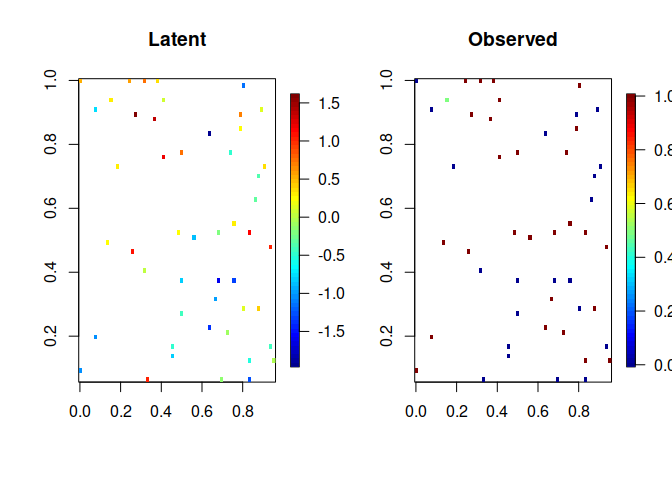par(defpar) Given the simulated data, we now can efficiently estimate the latent field by specifying the number of conditioning points $$m$$ described earlier. Interweaved ordering is best for 1D data while response-first (‘zy’) ordering is best for higher dimensions. m=10 if(spatial.dim==1){ vecchia.approx=vecchia_specify(locs,m) #IW ordering } else { vecchia.approx=vecchia_specify(locs,m,cond.yz='zy') #RF ordering } With the approximated covariance structure, we can calculate the posterior estimate for the latent field using the Vecchia-Laplace method and plot the result. Pure Laplace approximation is included for comparison; even with a small value for $$m$$, we can get a result similar to Laplace but with much lower cost. posterior = calculate_posterior_VL(z,vecchia.approx,likelihood_model=data.model, covparms = covparms) if (spatial.dim==1){ par(mfrow=c(1,1)) ord = order(locs) # order so that lines appear correctly y_limits = c(min(y, posterior$mean[ord]), max(y, posterior$mean[ord])) plot(locs[ord], y[ord], type = "l", ylim = y_limits ) lines(locs[ord], posterior$mean[ord], type = "l", col=3, lwd=3)
legend("bottomright", legend = c("Latent", "VL"), col= c(1,3), lwd=c(1,3))
} else if (spatial.dim==2){
dfpar = par(mfrow=c(1,2))
# ordering unnecessary; we are using a scatter plot rather than lines
quilt.plot(locs, y, main= "Truth")
quilt.plot(locs, posterior$mean, main= "VL m=10") par(defpar) }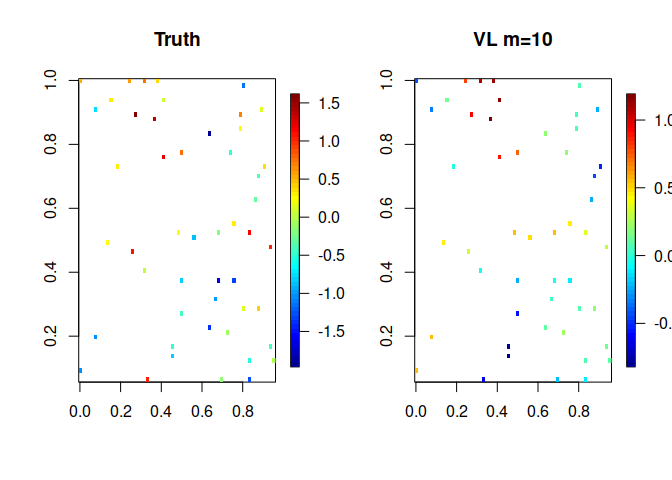### Non-Gaussian predictions Predictions are computed as before, using Vecchia-Laplace methods where needed ###### specify prediction locations ####### n.p=30^2 if(spatial.dim==1){ # 1-D case locs.pred=matrix(seq(0,1,length=n.p),ncol=1) } else { # 2-D case grid.oneside=seq(0,1,length=round(sqrt(n.p))) locs.pred=as.matrix(expand.grid(grid.oneside,grid.oneside)) # grid of pred.locs } n.p=nrow(locs.pred) ###### specify Vecchia approximation ####### vecchia.approx.pred = vecchia_specify(locs, m=10, locs.pred=locs.pred) ### carry out prediction preds = vecchia_laplace_prediction(posterior, vecchia.approx.pred, covparms) # plotting predicitions if (spatial.dim==1){ defpar = par(mfrow=c(1,1)) ord = order(locs) # order so that lines appear correctly plot(locs[ord], y[ord], type = "l", xlim=c(0,1.2), ylim = c(-1,3)) lines(locs, posterior$mean, type = "p", col=4, lwd=3, lty=1)
lines(locs.pred, preds$mu.pred, type = "l", col=3, lwd=3, lty=1) lines(locs.pred,preds$mu.pred+sqrt(preds$var.pred), type = "l", lty = 3, col=3) lines(locs.pred,preds$mu.pred-sqrt(preds$var.pred), type = "l", lty = 3, col=3) legend("topleft", legend = c("Latent", "VL: Pred", "VL: 1 stdev"), col= c(1,3,3), lwd=c(1,2,1), lty = c(1,1,3)) par(defpar) } else if (spatial.dim==2){ defpar = par(mfrow=c(1,2)) # ordering unnecessary; we are using a scatter plot rather than lines quilt.plot(locs, y, main= "True Latent", xlim = c(0,1), ylim = c(0,1), nx=64, ny=64) quilt.plot(locs.pred, preds$mu.pred,  main= "VL Prediction",nx = 30, ny=30)
par(defpar)
}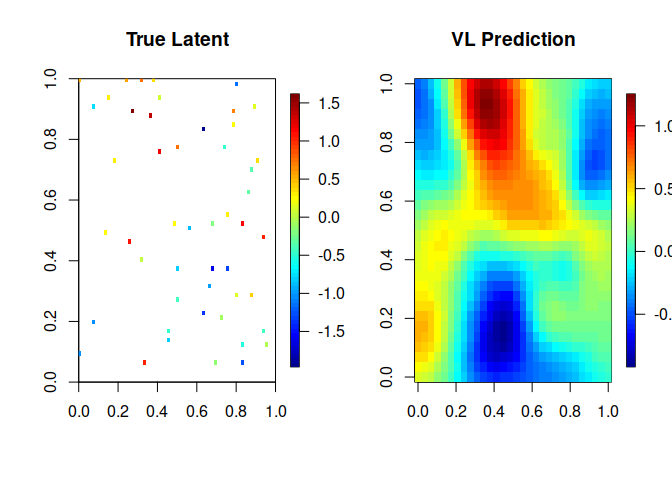### Parameter estimation

The likelihood of the data for a set of parameters can be computed efficiently using the command below.

vecchia_laplace_likelihood(z,vecchia.approx,likelihood_model=data.model,covparms = covparms)
#>  -34.23622

This can be used for parameter estimation by evaluating the likelihood over a grid of parameter values or in an iterative optimization method such as Nelder-Mead. Reparameterizing the parameters improves performance.

# currently set up for covariance estimation
vecchia.approx=vecchia_specify(locs, m=10, cond.yz = "zy") # for posterior
vecchia.approx.IW = vecchia_specify(locs, m=10) # for integrated likelihood
if (spatial.dim==1) vecchia.approx=vecchia.approx.IW

vl_likelihood = function(x0){
theta = exp(x0)
covparms=c(theta, theta, theta) # sigma range smoothness
prior_mean = 0 # can be a parameter as well
# Perform inference on latent mean with Vecchia Laplace approximation
vll = vecchia_laplace_likelihood(z,vecchia.approx, likelihood_model=data.model,
covparms, return_all = FALSE,
likparms = default_lh_params, prior_mean = prior_mean,
vecchia.approx.IW = vecchia.approx.IW)
return(-vll)

}
x0 = log(c(.07,1.88, 1.9))
vl_likelihood(x0)
# Issues with R aborting, maxit set to 1
res = optim(x0, vl_likelihood, method = "Nelder-Mead", control = list("trace" = 1, "maxit" = 1))
exp(res\$par[1:3])
vl_likelihood(x0)